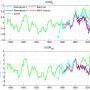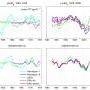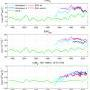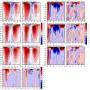(a) The 5-year running averaged monthly anomalies of global relative angular momentum, ΔMR, for the 5 reanalysis datasets. (b) Same as (a) but for ΔM200, the angular momentum (per unit pressure thickness) calculated at only 200 hPa level.(a) The least-square quadratic fit (dashed curve) to ΔMR for NCEP Reanalysis I, ERA-40, and 20CR for 1949-1978. (b) the “detrended” time series for the same period, with the quadratic curve removed from the time series. (c) Same as (a) but for 1979-2008 and with the addition of NCEP Reanalysis II and ERA-Interim.  (d) Same as (b) but for 1979-2008 and with the addition of NCEP Reanalysis II and ERA-Interim.(a) The global relative angular momentum, MR, with the long-term mean retained, for the five reanalysis datasets. (b) Same as (a) but for M200.  (c) Same as (a) but with the integration carried out only from 150-10hPa and 10°S-10°N to show the contribution from tropical upper atmosphere.(a) The summer (JJA)  climatology of zonal mean zonal wind from 1979-2008 for 20CR. (b) Same as (a) but for ERA-Interim. (c) The difference between 20CR and ERA-Interim, i.e., (b) minus (a). (d) is similar to (c) but for the difference between NCEP Reanalysis II and ERA-Interim.  (e)-(h) are similar to (a)-(d) but for winter (DJF). (i)-(l) are similar to (a)-(d) but for the annual mean.  (m) and (n) are the 1979-2008 linear trends for 20CR and ERA-Interim.  Contour intervals are 4 m s-1 for (a), (b), (e), (f), (i), and (j);  1 m s-1 for (c), (d), (g), (h), (k), (l), (m) and (n).